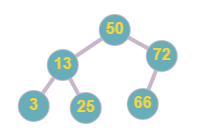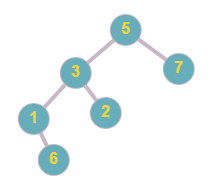# Insert Into A Binary Search Tree

Posted: 24 Mar, 2021
Difficulty: Easy

## PROBLEM STATEMENT

#### Note :

``````A binary search tree is a binary tree data structure, with the following properties :
a. The left subtree of any node contains nodes with the value less than the node’s value.
b. The right subtree of any node contains nodes with the value equal to or greater than the node’s value.
c. Right, and left subtrees are also binary search trees.
It is guaranteed that,
a. All nodes in the given tree are distinct positive integers.
b. The given BST does not contain any node with a given integer value.
``````

#### Example, below the tree, is a binary search tree.#### Below the tree is not a BST as node ‘2’ is less than node ‘3’ but ‘2’ is the right child of ‘3’, and node ‘6’ is greater than node ‘5’ but it is in the left subtree of node ‘5’.#### Input Format :

``````The first line contains an integer 'T' which denotes the number of test cases or queries to be run.

The first line of each test case contains elements of the tree in the level order form. The line consists of values of nodes separated by a single space. In case a node is null, we take -1 in its place.

The second line of each test case contains a positive integer value ‘val’, denoting the value of the node that is to be inserted in the given BST.

For example, the level order input for the tree depicted in the below image.
````````````50
13 72
3 25 66 -1
-1 -1 -1 -1 -1 -1
``````

#### Explanation :

``````Level 1 :
The root node of the tree is 50

Level 2 :
Left child of 50 = 13
Right child of 50 = 72

Level 3 :
Left child of 13 = 3
Right child of 13 = 25
Left child of 72 = 66
Right child of 72 =  ‘Null’

Level 4 :
All children are ‘Null’

The first not-null node(of the previous level) is treated as the parent of the first two nodes of the current level. The second not-null node (of the previous level) is treated as the parent node for the next two nodes of the current level and so on.
The input ends when all nodes at the last level are null(-1).
``````

#### Note :

``````The above format was just to provide clarity on how the input is formed for a given tree.

The sequence will be put together in a single line separated by a single space.

Hence, for the above-depicted tree, the input will be given as:
50 13 72 3 25 66 -1 -1 -1 -1 -1 -1 -1
``````

#### Output Format :

``````For each test case, the output will be “1” if you have returned the correct answer, else it will be “0”.

The output of each test case will be printed in a separate line.
``````

#### Note :

``````You do not need to input or print anything, and it has already been taken care of. Just implement the given function.
``````

#### Constraints :

``````1 <= T <= 5
0 <= N <= 3000
1 <= data <= 10 ^ 9
1 <= val <= 10 ^ 9

Where ‘N’ is the total number of nodes in the given binary tree, ‘data’ is the value of the nodes of the given binary tree, and ‘val’ represents the value of node that is to be inserted in the given tree.

For a single test case, all given ‘data’ and ‘val’ are distinct from each other.

Time Limit: 1sec
``````Approach 1

The rules of the binary search tree say if any node value is less than the root node value, then this node must lie on the left subtree of the root else will lie on the right subtree. We can think of a recursive solution by breaking the task into a subtask, as any node can be either on the left subtree or right subtree of the root node. In BST we can find which subtree (left/right) to follow next by comparing the given value and root node’s value.

For example, if the given value is ‘3’ and the root node’s value is ‘5’ the node will be inserted in the left subtree of the root node as ‘3 < 5’. Similarly, if the given value is greater than the root node’s value we can assure that the node will insert on the right subtree of the root node. For a given tree we can find a required position by recursively calling the function to find the position in left subtree or right subtree after comparing values of nodes. When we reach a subtree whose root node is ‘Null’, then this means that we have reached the required position.

## Algorithm :

• Base case - If the root is NULL, return a tree node with a value same as the given value.
• If the given value is greater than the value of the root node, then recursively call the function for the right subtree and update the right subtree with the returned tree from the recursive function.
• Else, call the function for the left subtree and update the left subtree with the returned tree from the recursive function.
• Return root node.